# Floating point and Double Data Type

Numbers can be represented using Integer data types in C++. But some numbers like real numbers cannot be stored like integers because there is a decimal part associated with the real numbers.

C++ programming language has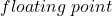and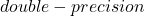data type to represent real numbers.

A real number is declared using keyword –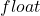or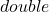. The main difference betweenandis the size. The size of float is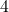bytes or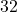bits, where the size oftype is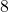bytes or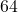bits. There is a long version of thedata type which is about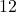bytes or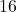bytes in size.

## Computer Representation of Floating Point

The real numbers are represented in scientific notation (or exponential notation) because it is easier to perform arithmetic involving real values.

The Exponential Notation

The exponential notation has two parts –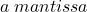and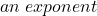.  The equation to represent thenumbers in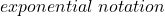is shown below.

\begin{aligned}&\pm M * 10^E\\ \\ &where \\ \\ &0 \leq M \leq 10\end{aligned}

For example, suppose you want to represent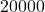in exponential notation then it becomes

\begin{aligned}&2.0 \cdot 10^4\\ \\&where \\ \\ &0 \leq 2 \leq 10\end{aligned}

If you want to represent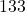in scientific notation, then

\begin{aligned}&1.33 \cdot 10^3\\ \\&where \\ \\&0 \leq 1.33 \leq 10\end{aligned}

The number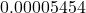can be represented as

\begin{aligned}&5.454 \cdot 10^{-5} \\ \\&where\\ \\&0 \leq 5.454 \leq 10\end{aligned}

The table isolates the different parts of the examples given above.

The above notation is suitable for human, but the computer needs a binary representation ofnumbers and that too, in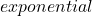format.

Since we already know thatbytes orbit is required to store a floating point number in a computer. The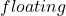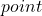number is divided into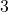parts –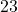bits for the mantissa,bit for sign, andbit for exponents.

The sign bit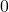means positive number andmeans a negative number.

The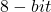exponent can store values between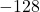to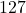.

The computer representation of exponential notation is:

\begin{aligned}&(b_0. b_1 b_2 b_3 \cdots ) \cdot 2^E\\ \\&where \\ \\&b_{0} = 1\end{aligned}

This representation is called the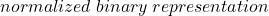.

## Declaring Floating Type and Double Type

Declaring a floating type and double data type variabe in a C program is similar.

float PI  3.14;
double radius 5,33;

There is little difference betweenandthough they are represented in the same way in a computer. The double precision is longer than the float in terms of allowing the real part of a floating number.

\begin{aligned}&3.244440\\ \\&3.244440000000000 \hspace{3px}(double \hspace{3px} is \hspace{3px} has \hspace{3px} longer \hspace{3px} decimal \hspace{3px} part )\end{aligned}